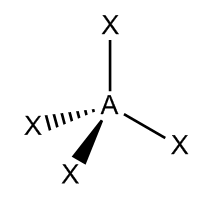# Problem: How many structures are possible for a tetrahedral molecule with a formula of AHXYZ?How many structures are possible for a tetrahedral molecule with a formula of AX 3Y?

###### FREE Expert Solution

In a tetrahedral molecular geometry, a central atom is located at the center with four substituents that are located at the corners of a tetrahedron and are approximately equally spaced with bond angles of approximately 109.5o

A molecule AX4 where A is the central atom would look like the illustration below.The dashes and wedge bonds are shown to illustrate the position in space of the elements.

88% (146 ratings)###### Problem Details

How many structures are possible for a tetrahedral molecule with a formula of AHXYZ?

How many structures are possible for a tetrahedral molecule with a formula of AX 3Y?